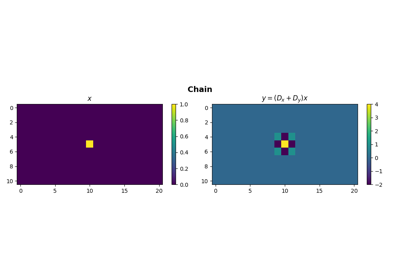# pylops.Kronecker¶

class pylops.Kronecker(Op1, Op2, dtype='float64')[source]

Kronecker operator.

Perform Kronecker product of two operators. Note that the combined operator is never created explicitly, rather the product of this operator with the model vector is performed in forward mode, or the product of the adjoint of this operator and the data vector in adjoint mode.

Parameters: Op1 : pylops.LinearOperator First operator Op2 : pylops.LinearOperator Second operator dtype : str, optional Type of elements in input array.

Notes

The Kronecker product (denoted with $$\otimes$$) is an operation on two operators $$\mathbf{Op}_1$$ and $$\mathbf{Op}_2$$ of sizes $$\lbrack n_1 \times m_1 \rbrack$$ and $$\lbrack n_2 \times m_2 \rbrack$$ respectively, resulting in a block matrix of size $$\lbrack n_1 n_2 \times m_1 m_2 \rbrack$$.

$\begin{split}\mathbf{Op}_1 \otimes \mathbf{Op}_2 = \begin{bmatrix} \text{Op}_1^{1,1} \mathbf{Op}_2 & \ldots & \text{Op}_1^{1,m_1} \mathbf{Op}_2 \\ \vdots & \ddots & \vdots \\ \text{Op}_1^{n_1,1} \mathbf{Op}_2 & \ldots & \text{Op}_1^{n_1,m_1} \mathbf{Op}_2 \end{bmatrix}\end{split}$

The application of the resulting matrix to a vector $$\mathbf{x}$$ of size $$\lbrack m_1 m_2 \times 1 \rbrack$$ is equivalent to the application of the second operator $$\mathbf{Op}_2$$ to the rows of a matrix of size $$\lbrack m_2 \times m_1 \rbrack$$ obtained by reshaping the input vector $$\mathbf{x}$$, followed by the application of the first operator to the transposed matrix produced by the first operator. In adjoint mode the same procedure is followed but the adjoint of each operator is used.

Attributes: shape : tuple Operator shape explicit : bool Operator contains a matrix that can be solved explicitly (True) or not (False)

Methods

 __init__(Op1, Op2[, dtype]) Initialize this LinearOperator. adjoint() Hermitian adjoint. apply_columns(cols) Apply subset of columns of operator cond([uselobpcg]) Condition number of linear operator. conj() Complex conjugate operator div(y[, niter, densesolver]) Solve the linear problem $$\mathbf{y}=\mathbf{A}\mathbf{x}$$. dot(x) Matrix-matrix or matrix-vector multiplication. eigs([neigs, symmetric, niter, uselobpcg]) Most significant eigenvalues of linear operator. matmat(X) Matrix-matrix multiplication. matvec(x) Matrix-vector multiplication. rmatmat(X) Matrix-matrix multiplication. rmatvec(x) Adjoint matrix-vector multiplication. todense([backend]) Return dense matrix. toimag([forw, adj]) Imag operator toreal([forw, adj]) Real operator tosparse() Return sparse matrix. trace([neval, method, backend]) Trace of linear operator. transpose() Transpose this linear operator.

## Examples using pylops.Kronecker¶Operators concatenation